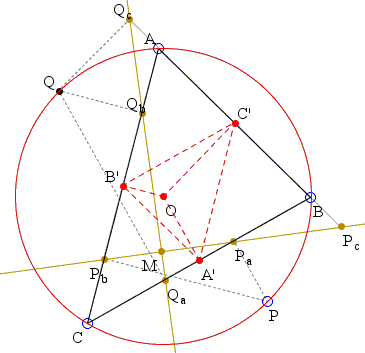## Simsons of Diametrically Opposite PointsWhat Is This About? A Mathematical Droodle

ExplanationLet P lie on the circumcircle of triangle ABC. Let Q be the point on the circumcircle diametrically opposite P. Then the Simson lines of P and Q are perpendicular. As P changes, the points of intersection of the two simsons traces the 9-point circle of ABC.

If you change one of the vertices A, B, or C, the intersection point still traverses a circle.

Hubert Shutrick sent me the following proof. Let Pa, Pb, Pc and Qa, Qb, Qc be the feet of the perpendiculars from P and Q onto BC, AC, AB.In the diagram, angles CPaP and CPbP are right which makes quadrilateral CPPaPb cyclic. It follows that the inscribed angles CPaPb and PPaPb are equal. A similar argument applies to show that quadrilateral CQQbQa is also cyclic and that angles QCQb and QQaQb are equal.

Now, angles CPPb and QCQb have perpendicular sides and are therefore equal. It follows that

∠CPaPb = ∠QQaQb

But one pair of their sides, viz., CPa and QQA are orthogonal which implies that this is also true for the second pair:

PaPb ⊥ QaQb.

This proves that the simsons of P and Q are indeed orthogonal.Let A'B'C' be the medial triangle of ΔABC.ĀSince the perpendicular to BC at A' passes through the circumcenter of ABC, A' is also the midpoint of PaQa and the right angle gives that A'M = A'Pa so there is an angle θ at M also.Ā By the same token there is the same angle φ at M and Pc:

∠MPcC' = ∠MC'Pc.

However,

Ā

∠A'B'C' = ∠ABC = θ + φ = ∠A'MC',

so that M is on the circumcircle of ΔA'B'C', the nine point circle of ABC. Conversely, given a point M such that ∠A'B'C' =Ā ∠A'MC, we have candidates for Pa and Qa whose distances from A' are A'M. From them we construct two diagonally opposite pairs P, Q and P', Q' on the circumcircle of ABC, where, in the diagram, the other candidate for P' for P would be above the line BC.Ā The Simson lines of each pair intersect at right angles and pass through Pa and Qa and so their points of intersection are the points of intersection of the circle with diameter PaQa with the nine point circle.ĀOne of these must be the given M. We have ignored the interchange of P with Q that gives the same point M and the fact that the nine point circle is described twice as P goes round the circumcircle. Q(uite) E(asily) D(one)

### Note

Hubert Shutrick found a generalization of this property to the case of two arbitrary points P and Q.

### References

1. F. G.-M., Exercise de Géométrie, Éditions Jacques Gabay, 1991, p. 330### Simson Line - the simson

• Simson Line: Introduction
• Simson Line
• Three Concurrent Circles
• 9-point Circle as a locus of concurrency
• Miquel's Point
• Circumcircle of Three Parabola Tangents
• Angle Bisector in Parallelogram
• Simsons and 9-Point Circles in Cyclic Quadrilateral
• Reflections of a Point on the Circumcircle
• Simson Line From Isogonal Perspective
• Pentagon in a Semicircle
• Simson Line in Disguise
• Two Simsons in a Triangle
• Carnot's Theorem
• A Generalization of Simson Line